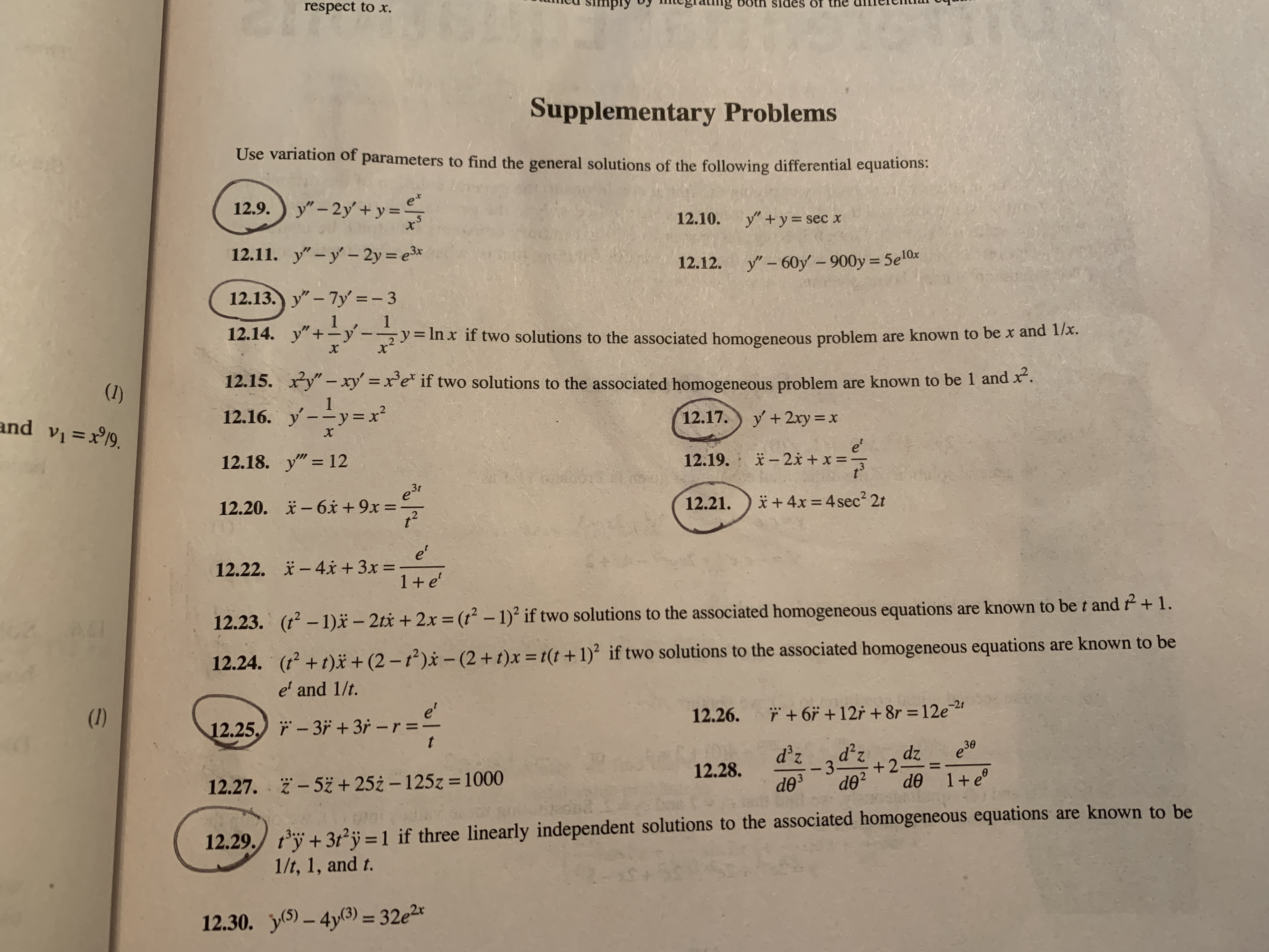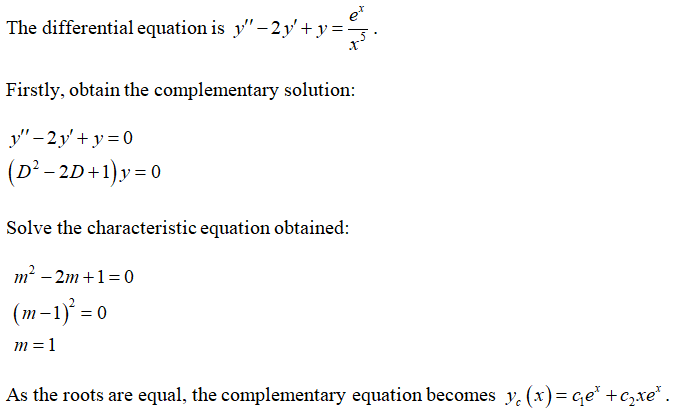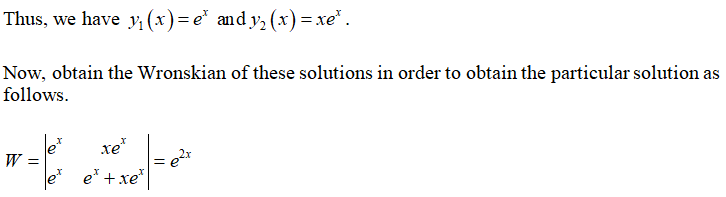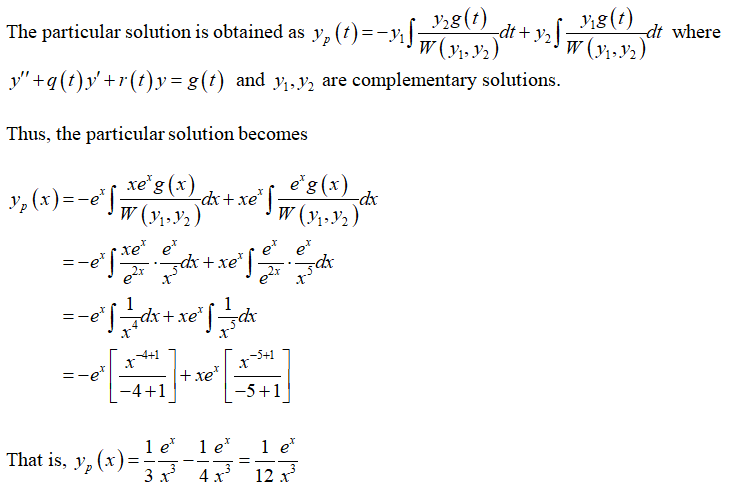# respect to x.ngsides ofSupplementary ProblemsUse variation of parameters to find the general solutions of the following differential equations:12.9.) y" - 2y' + y =et12.10.y" +y= sec x12.11. y" -y'- 2y = e3*12.12.y" – 60y' – 900y = 5e10x%3D12.13.) y"- 7y' =- 312.14. y"+y-y = In x if two solutions to the associated homogeneous problem are known to be x and 17x.12.15.xy"- xy' x'e* if two solutions to the associated homogeneous problem are known to be 1 and x".(1)12.16. y--y=x²and v =x19.y +2xy = x12.17.%3De'* - 2x + x =-12.18. y" = 12%3D12.19.12.20.й — 6х +9х:*+ 4x = 4 sec? 2t12.21.e'* - 4x + 3x =12.22.%3D1+e'(t – 1)ä – 2tx + 2x = (t – 1) if two solutions to the associated homogeneous equations are known to be t and + 1.12.23.%3D(t2 +t)ï + (2 – t²)x – (2 +t)x =t(t+1)² if two solutions to the associated homogeneous equations are known to be12.24.e' and 1/t.(1)e'12.25.) - 3ii + 3r -r =-ř + 6ř +12r + 8r = 12e212.26.%3Dd'z3e30dz+2-de 1+ e°12.28.- 52 + 25ż – 125z = 1000%3D12.27.d0²do312.29. t°ÿ + 3t²j = 1 if three linearly independent solutions to the associated homogeneous equations are known to be1/t, 1, and t.y(5) – 4y(3) = 32e2r12.30.%3D/2

Question
8 views

Question #12.9 from the image. Differential Equationshelp_outlineImage Transcriptioncloserespect to x. ng sides of Supplementary Problems Use variation of parameters to find the general solutions of the following differential equations: 12.9.) y" - 2y' + y = et 12.10. y" +y= sec x 12.11. y" -y'- 2y = e3* 12.12. y" – 60y' – 900y = 5e10x %3D 12.13.) y"- 7y' =- 3 12.14. y"+y- y = In x if two solutions to the associated homogeneous problem are known to be x and 17x. 12.15. xy"- xy' x'e* if two solutions to the associated homogeneous problem are known to be 1 and x". (1) 12.16. y--y=x² and v =x19. y +2xy = x 12.17. %3D e' * - 2x + x =- 12.18. y" = 12 %3D 12.19. 12.20. й — 6х +9х: *+ 4x = 4 sec? 2t 12.21. e' * - 4x + 3x = 12.22. %3D 1+e' (t – 1)ä – 2tx + 2x = (t – 1) if two solutions to the associated homogeneous equations are known to be t and + 1. 12.23. %3D (t2 +t)ï + (2 – t²)x – (2 +t)x =t(t+1)² if two solutions to the associated homogeneous equations are known to be 12.24. e' and 1/t. (1) e' 12.25.) - 3ii + 3r -r =- ř + 6ř +12r + 8r = 12e2 12.26. %3D d'z 3 e30 dz +2- de 1+ e° 12.28. - 52 + 25ż – 125z = 1000 %3D 12.27. d0² do3 12.29. t°ÿ + 3t²j = 1 if three linearly independent solutions to the associated homogeneous equations are known to be 1/t, 1, and t. y(5) – 4y(3) = 32e2r 12.30. %3D /2 fullscreen
check_circle

Solution:Compute Wronskian:Obtain the particular solution:...

### Want to see the full answer?

See Solution

#### Want to see this answer and more?

Solutions are written by subject experts who are available 24/7. Questions are typically answered within 1 hour.*

See Solution
*Response times may vary by subject and question.
Tagged in